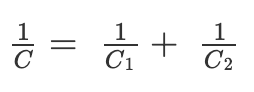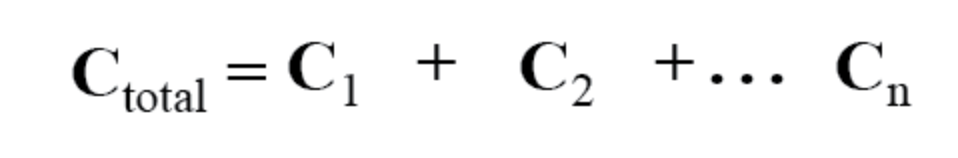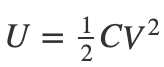# How do you solve a capacitor problem?

In a given capacitor circuit, we will mostly be asked to identify the total capacitance of the circuit, the energy stored in the circuit or the voltage across the capacitor. Each of these parameters can be found out using specific formulas by following some rules.

## Identifying the Total Charge in a Given Circuit

The total charge in a circuit depends on the total capacitance and total voltage. This relationship is equated as follows:

Q = C/V

By rearranging the equation, we can identify the value of the capacitance as:

C = Q/V

## Identifying the total capacitance of a given circuit

Capacitors in a circuit can either be connected in series or parallel based on the requirement.

When capacitors are connected in series, the total capacitance is calculated using the following formula:When capacitors are connected in parallel, the total capacitance in the circuit is calculated using the following formula:## Identifying the Energy Stored in a Capacitor

The energy stored in a capacitor is the electric potential energy and is related to the voltage and charge on the capacitor.

The energy stored in the capacitor is given by the following formula:where C is the capacitance and V is the voltage.(1)(0)